# How to export designed IIR/FIR filters to Matlab

For many IoT sensor measurement applications, an IIR or FIR filter is just one of the many components needed for an algorithm. This could be a powerline interference canceller for a biomedical application or even a simpler DC loadcell filter. In many cases, it is necessary to integrate a filter into a complete algorithm in another domain.

Matlab is a well-established numerical computing language developed by the Mathworks that allows for the design of algorithms, matrix data manipulations and data analysis. The product offers a broad range of algorithms and support functions for signal processing applications, and as such is very popular amongst many scientists and engineers worldwide.

## ASN Filter Designer automatic code generator for Matlab

The ASN Filter Designer greatly simplifies exporting a designed filter to Matlab via its automatic code generator. The code generator supports all aspects of the ASN Filter Designer, allowing for a complete design comprised of H1, H2 and H3 filters and math operators to be fully integrated with an algorithm in Matlab.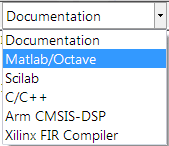The Matlab code generator can be accessed via the filter summary options (as shown on the right). Selecting this option will automatically generate a Matlab `.m` file based on the current design.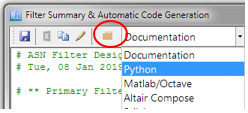A convenient shortcut to the relevant Framework files and examples is available in the Filter summary toolbar via the folder symbol (see left).

Using the three framework files, you may build a demo of your choice based on the exported filter(s) specifications. The framework supports both Real and Complex filters in floating point only. In order to use the generated code in Matlab without the need for Signal Processing Toolbox, the following three framework files are provided in the ASN Filter Designer’s `\Matlab` directory:

`ASNFDMatlabFilterData.m`
`ASNFDMatlabImport.m`
`ASNFDFilter.m`

These framework files do not have any special Matlab toolbox dependences, and the example script `ASNFDMatlabDemo.m` demonstrates the simplicity with which the framework can be integrated into your application for your designed filter. Several example filters generated via the automatic code generator are given within `ASNFDMatlabDemo.m` in order to get you going!

## Comparing the results to Matlab’s Signal Processing Toolbox

It’s sometimes informative to compare the results of the ASN Filter Designer’s DSP library functions to that of Matlab’s Signal Processing Toolbox.

Designing an IIR Chebyshev Type I filter with the following specifications:

 Fs: 500Hz Passband frequency: 0-25Hz Type: Lowpass Method: Chebyshev Type I Stopband attenuation @ 125Hz: ≥ 80 dB Passband ripple: ≤ 0.1dB Order: 5

Graphically entering the specifications into the ASN Filter Designer, and fine tuning the design marker positions, the tool automatically designs the filter as a Biquad cascade. Notice that the tool automatically finds the required filter order, and in essence – automatically produces the filter’s exact technical specification!

The frequency response of a 5th order IIR Chebyshev Type I lowpass filter meeting the specifications is shown below: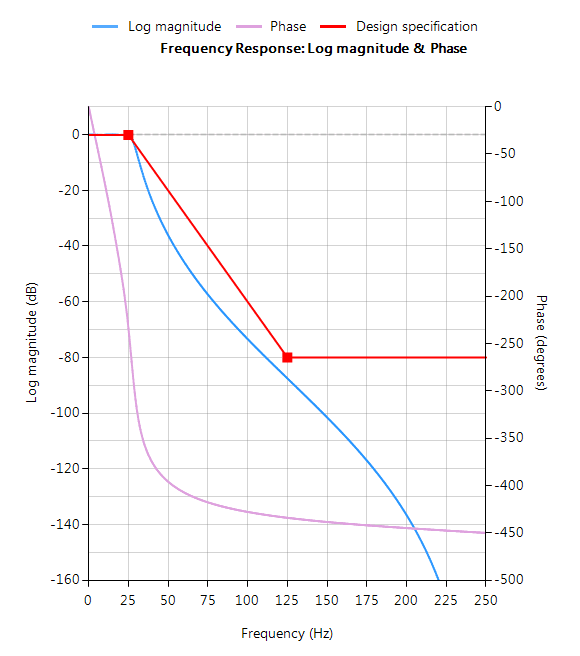The resulting filter coefficients are: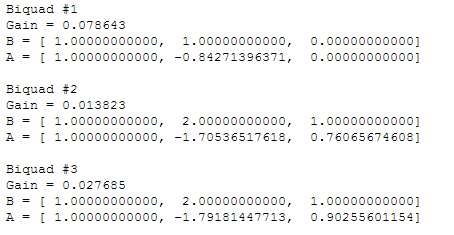Designing the same filter in Matlab using Signal Processing Toolbox:

```Fs=500;
Rp=0.1;
Rs=80;
F=2*[25,125]/Fs;

[N,Wn]=cheb1ord(F(1),F(2),Rp,Rs)
[z, p, k] = cheby1(N,Rp,Wn,'low'); % design lowpass

[sos,g]=zp2sos(z,p,k,'up')  % generate SOS form
```

Running the script, we get the following, where each row of `sos` is a biquad arranged as: ` b0 b1 b2 a0 a1 a2 `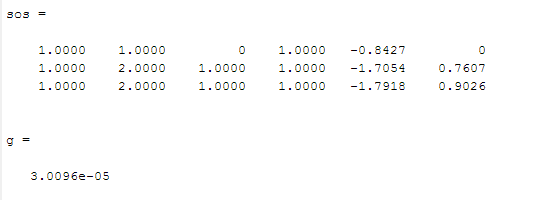Analysing both sets of numerator and denominator coefficients, we get exactly the same result! But what about the gain? Matlab outputs a net gain, `g = 3.0096e-05` but the ASN Filter Designer optimally assigns a gain to each biquad. Thus, combining the biquad section gains, i.e. ` 0.078643, 0.013823 ` and `0.027685` results in a net gain of `3.0096e-05`, which is exactly the same net gain as Matlab!

Conclusion: the ASN Filter Designer’s DSP IIR library functions completely match Matlab’s Signal Processing Toolbox results!!

The complete automatically generated code is shown below, where it can be seen that the biquad gains have been pre-multiplied with the feedforward coefficients.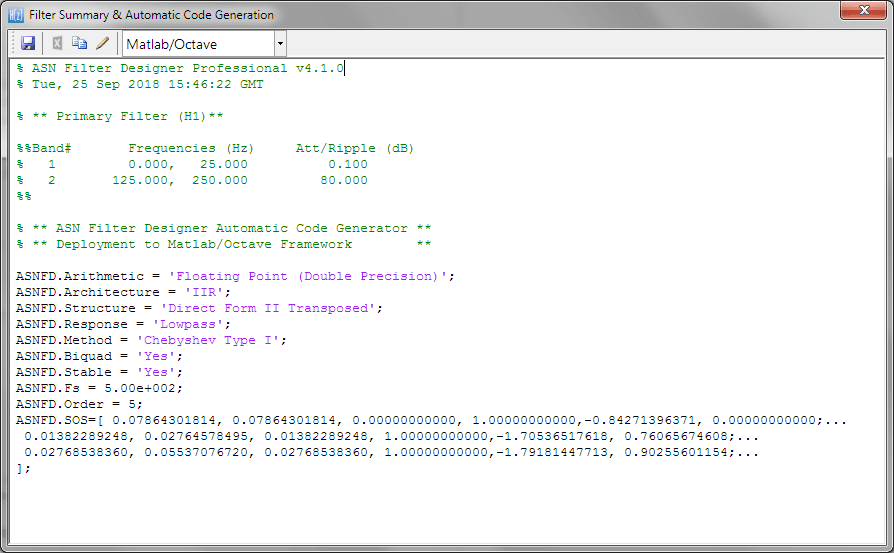## Using the generated code with Signal Processing Toolbox

If you have Signal Processing Toolbox installed, then you may directly use the generated coefficients given in SOS with the `sosfilt()` command, e.g.

```Clear all;

ASNFD_SOS=[ 0.07864301814, 0.07864301814, 0.00000000000, 1.00000000000,-0.84271396371, 0.00000000000;...
0.01382289248, 0.02764578495, 0.01382289248, 1.00000000000,-1.70536517618, 0.76065674608;...
0.02768538360, 0.05537076720, 0.02768538360, 1.00000000000,-1.79181447713, 0.90255601154;...
];

y=sosfilt(ASNFD_SOS, x); %  x is your input data

plot(x,y); % plot results
```

As seen, it is as simple as copying and pasting the filter coefficients from the ASN Filter Designer’s filter summary into a Matlab script.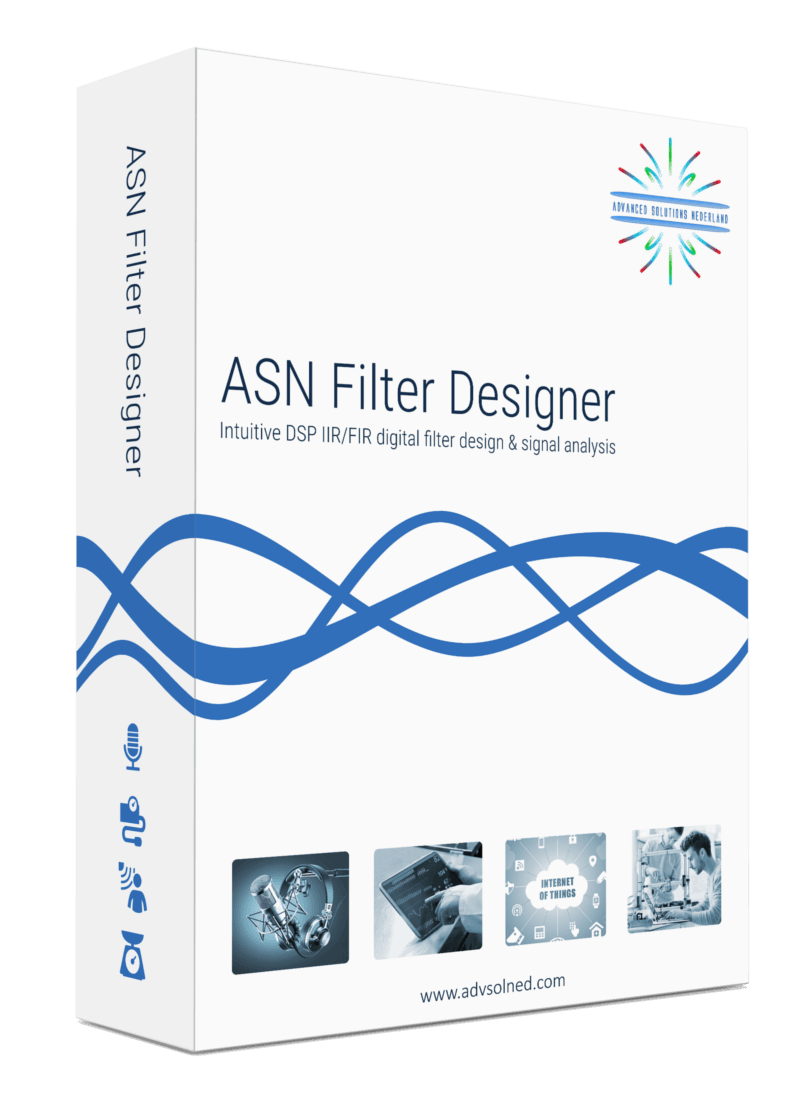Tags:
0 replies